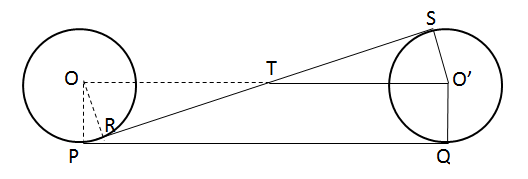# CAT Quantitative Aptitude Questions | Geometry Questions for CAT - Triangles

###### CAT Questions | CAT Geometry Questions | Circles

The question is from the topic circles. It discusses about two circles and their direct and indirect tangents. CAT Geometry questions are heavily tested in CAT exam. Make sure you master Geometry problems. We have to find out the length of the direct tangent given the length of the indirect tangent.

Question 38: Two circles of radius 5 cm have a direct tangent PQ and an indirect tangent RS. Find the length of PQ if RS = 24 cm.

1. 29 cm
2. 13 cm
3. 26 cm
4. Cannot be determined due to lack of information

## Best CAT Coaching in Chennai

#### CAT Coaching in Chennai - CAT 2021Online Batches Available Now!

##### Method of solving this CAT Question from Circles:Draw the diagram, then you are through.Since, OP = PQ and OP⊥PQ and O’Q⊥PQ
PQ = OO’
Now in △ORT and △O’ST
∠ORT = ∠O’ST
∠OTR = ∠STO’
∴ △ORT is similar to O’ST (AA)
∴ OR/OS = RT/TS => RT/TS = 5/5 = 1
=> RT = TS
Given RS = 24 cm
∴ RT = TS = 12cm
∴ OT = √((OR)2 + (RT)2 ) = √(52 + 22 ) = 13 cm = O’T
∴ OO’ = PQ = 13 + 13 = 26 cm

The question is "Find the radius of the circle"

##### Hence, the answer is 26 cm.

Choice C is the correct answer.

###### CAT Coaching in ChennaiCAT 2021Enroll at 49,000/- 44,000/-

Online Classroom Batches Starting Now!

###### Best CAT Coaching in ChennaiPrices slashed by Rs 4000/-

Attend a Demo Class

## CAT Preparation Online | CAT Geometry questions Videos On YouTube

#### Other useful sources for Geometry Question | Geometry Triangles Circles Quadrilaterals Sample Questions

##### Where is 2IIM located?

2IIM Online CAT Coaching
A Fermat Education Initiative,
58/16, Indira Gandhi Street,
Kaveri Rangan Nagar, Saligramam, Chennai 600 093

##### How to reach 2IIM?

Phone: (91) 44 4505 8484
Mobile: (91) 99626 48484
WhatsApp: WhatsApp Now
Email: prep@2iim.com